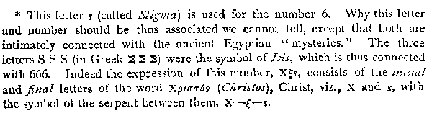In Search of the Beast 666 (aka Anti-Christ)
 Greek Numerology Enter a Greek name, word, or phrase using English Equivalent transliterated characters: Try also Jesus (IHSOUS in transliterated characters). The difference even numerically between Christ and Anti-Christ is not great. Beware! "Pride goeth before destruction" Pr 16:18 Note: The number value of each letter using the Greek Numerology method, as in the Bible, is a quantitative method. Numbers are not reduced to a single digit to find the qualitative value and vowels and consonants are not separated as in modern numerological methods.
 Greek Letter Numerical Value English Equivalent Sound alpha 1 A a beta 2 B b gamma 3 G g (hard) delta 4 D d epsilon 5 E e (short) zeta 7 Z z eta 8 H e (long) theta 9 Q th iota 10 I i kappa 20 K k lamda 30 L l mu 40 M m nu 50 N n xi 60 X x omicron 70 O o (short) pi 80 P p rho 100 R r sigma 200 S s tau 300 T t upsilon 400 U u phi 500 V ph chi 600 C ch psi 700 J ps omega 800 W o (long)Missing letters? Missing numbers?
Stigma aka Digamma (
\$= 6) Koppa (& =90) Sampsi (" =900)

The Greek Alphabet

The Greek letters were 24 and the required number,27, was made up by using the final "s" or \$(called Stigma) for 6, and adding two arbitrary symbols called respectively Koppa, for 90, and Sampsi, for 900.

 Alpha=1 Iota=10 Rho=100 Beta=2 Kappa=20 Sigma=200 Gamma=3 Lambda=30 Tau=300 Delta=4 Mu=40 Upsilon=400 Epsilon=5 Nu=50 Phi=500 Stigma=6 Xi=60 Chi=600 Zeta=7 Omicron=70 Psi=700 Eta=8 Pi=80 Omega=800 Theta=9 Koppa=90 Sampsi=900Search the King James Version of the Bible